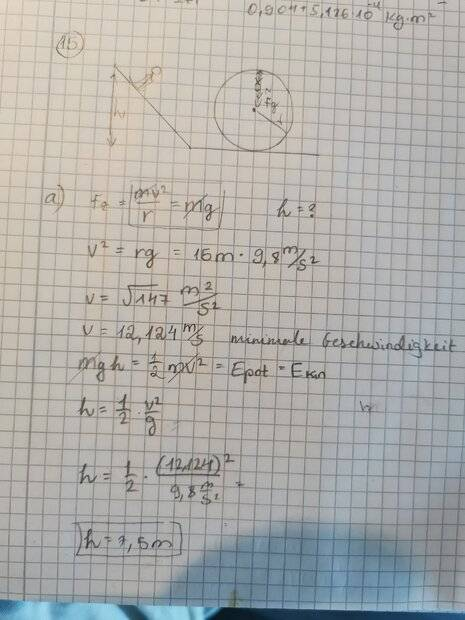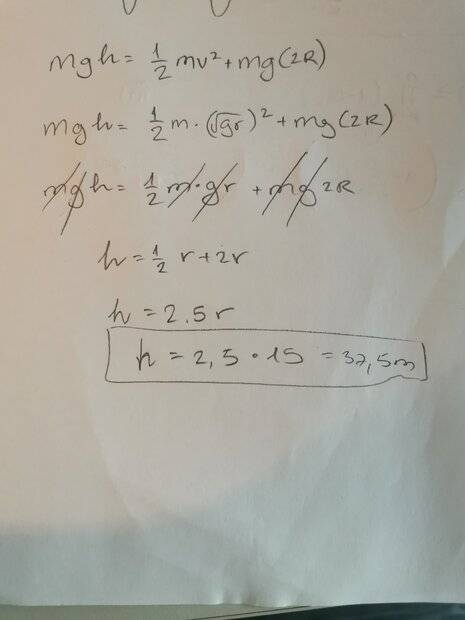# Loop problem with skier on ramp going into a loop-the-loop

• PainlessPeach
In summary, the conversation is about a physics assignment on a skier going through a loop with a ramp of certain height and radius. The first question asks how high the ramp should be for the skier to get through the loop if they are held by a safety rail system. The second question asks for the height of the ramp if there is no safety rail system. The difference between the two cases is that in the first case, the skier is held in place, while in the second case, they are not. The student has attempted to solve the problem in two different ways, using potential and kinetic energy and considering the force of gravity and centripetal force. They have asked for clarification on their answers and have been directed tof

#### PainlessPeach

Homework Statement
Loop Problem and Energy conservation
Relevant Equations
Fc=mv/2r , mg, E= 0.5mv^2, E= mgh
I have an assignment in physics and I can't understand the difference between the 2 questions. That's the question

A skier with mass m=70 kg stands on a ramp of height h. At the foot of the ramp, there is a loop with a radius of r=15 m. When entering the ramp, the speed is v1=0 m/s.

a) For test purposes, the skier is also held in the loop by a rail system so that he cannot fall off if his speed is too low. How high does the ramp have to be at least so that he can get through the loop in this case? Here I considered the loop as a hill that he needs to get through so I got that ramp should be 30m, since the radius is 30m.

b) Normal operation of the loop takes place without a safety rail system. In this case, how high does the ramp have to be so that the skier can get through the loop without falling off? Here I thought that the force of gravity must be equal to the centripetal force. So I got that h is 7.5, that means half of the r.

I can't understand what difference that makes if the person is held by a rail system or not. I tried to solve it in 2 ways considering the potential and kinetic energy, so I'm not sure that my answer is correct. It would be really helpful is someone could say that what I thought makes sense.
Thank you

•PainlessPeach
Homework Statement: Loop Problem and Energy conservation
Relevant Equations: Fc=mv/2r , mg, E= 0.5mv^2, E= mgh

the force of gravity must be equal to the centripetal force
Right idea, suggesting that you have understood the difference between the two cases, but you somehow arrived at a lower number. Did you get confused between the velocity at the bottom of the loop and the velocity at the top?

I can't understand what difference that makes if the person is held by a rail system or not.
So, how did you get different answers ?
a) [...] Here I considered the loop as a hill that he needs to get through so I got that ramp should be 30m, since the radius is 30m.
The question states that the radius of the loop is ##15m##. Does that change your answer ?
b) [...] So I got that h is 7.5, that means half of the r.
To be clear, your answer says that starting from a height of 7.5 somethings above ground will enable the skier to travel through a loop with a 15(or maybe 30?)m radius ?

Hey, thanks for reaponding. I just assumed that the calculation should be different if they asked 2 different things..Right idea, suggesting that you have understood the difference between the two cases, but you somehow arrived at a lower number. Did you get confused between the velocity at the bottom of the loop and the velocity at the top?
I didn't consider the velocity at the bottom, but the minimal velocity. If I understood correctly..
According to our rules, to receive help, you need to show some credible effort towards answering the question(s). How about showing us exactly what you did to get the answer you got?

According to our rules, to receive help, you need to show some credible effort towards answering the question(s). How about showing us exactly what you did to get the answer you got?

Oh sorry I will post my way now. Let me know if images are fine or maybe I should write the equations here aswell. And That was my third assumption:I didn't consider the velocity at the bottom, but the minimal velocity. If I understood correctly..

Oh sorry I will post my way now. Let me know if images are fine or maybe I should write the equations here aswell. And That was my third assumption:
View attachment 327306
That looks fine. Did you forget to add the 2r in post #1?

Wrt images, they should really only be used for diagrams and textbook extracts, but your writing is quite clear and your working is organised.

Let me know if images are fine or maybe I should write the equations here as well.
You should write the equations, using ## \LaTeX ##, see https://www.physicsforums.com/help/latexhelp/. That way people trying to help you can choose "Reply" and edit what you have posted. It also avoids confusion (is that a "2" or a "7" in your final answer)?

In your first post you gave the answer to (b) as 7.5m but now you have reached 37.5m, which is correct. Did you forget the ## mg(2r) ## term first time round?

You should write the equations, using ## \LaTeX ##, see https://www.physicsforums.com/help/latexhelp/. That way people trying to help you can choose "Reply" and edit what you have posted. It also avoids confusion (is that a "2" or a "7" in your final answer)?

In your first post you gave the answer to (b) as 7.5m but now you have reached 37.5m, which is correct. Did you forget the ## mg(2r) ## term first time round?
thanks for your reply, yes I tried to calculate it again with the ## mg(2r) ## term and got 37.5 m. This refers to (b) when the skier has no securing system. But I'm still not sure about how to solve (a) considering the rail.

thanks for your reply, yes I tried to calculate it again with the ## mg(2r) ## term and got 37.5 m. This refers to (b) when the skier has no securing system. But I'm still not sure about how to solve (a) considering the rail.
Without the rail, what will happen to the skier with a ramp a bit less than 37.5m?

If there is no rail, the skier must have enough speed for to hold him against the loop. If he tries to attempt the loop from less then 37.5m, he would approach the top half of the loop and just fall to the ground?

thanks for your reply, yes I tried to calculate it again with the ## mg(2r) ## term and got 37.5 m. This refers to (b) when the skier has no securing system. But I'm still not sure about how to solve (a) considering the rail.
Now I am as confused as you are. With a rail in place the skier only needs to reach the top of the loop as his velocity reaches 0: if we ignore the fact that his centre of mass is not at the point of contact with the ground/loop/rail the solution to part (a) is 30 m as you wrote in your OP:

a) For test purposes, the skier is also held in the loop by a rail system so that he cannot fall off if his speed is too low. How high does the ramp have to be at least so that he can get through the loop in this case? Here I considered the loop as a hill that he needs to get through so I got that ramp should be 30m, since the radius is 30m.

If there is no rail, the skier must have enough speed for to hold him against the loop. If he tries to attempt the loop from less then 37.5m, he would approach the top half of the loop and just fall to the ground?

Now I am as confused as you are. With a rail in place the skier only needs to reach the top of the loop as his velocity reaches 0: if we ignore the fact that his centre of mass is not at the point of contact with the ground/loop/rail the solution is trivial.
Is reaching the top of the loop with zero velocity a guarantee that the skier will lose contact with the loop at the top and not at some point before the top? Assume that the skier has just enough speed at the bottom to reach the top with zero speed and calculate the angle, with respect to the vertical, at which the normal force on the skier goes to zero. Is this angle zero?

Hint: Whatever trajectory the skier follows, it is symmetric about the vertical diameter of the loop and that includes his speed assuming of course no losses.

•haruspex
Welcome, @PainlessPeach !Assuming the loop has rails for both cases, try solving both problems in reverse:
- Starting from top of the loop at a zero velocity, how high on the ramp the skater will reach?
- Starting from top of the loop at the calculated minimum velocity, how high on the ramp the skater will reach?